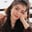Related Tags

java
communitycreator

# How to use nextUp(double d) in JavaFouzia Bashir

The static nextUp() method returns the floating-point value adjacent to N (argument value), but in the direction of positive infinity.

### Syntax

static double nextUp(double N)


### Parameters

N: The initial floating-point value

### Return value

This function will return the adjacent floating-point value in the direction of positive infinity (+∞).

### Special cases

Here are some special cases regarding nextUp():

• If the argument is NaN, then the result will also be NaN.
• If the argument is positive infinity, we will get positive infinity as a result.
• If we give zero as an argument, then the output will be Double.MIN_VALUE.

### Code

The following code illustrates how to use Math.nextUp().

import java.lang.*;
public class EdPresso {
public static void main(String[] args) {
// initialize the variables with double values
double n1 = 91868.321;
double n2 = 786;
// printing the next floating-point values directed towards positive infinity
System.out.println("Math.nextUp(" + n1 + ")= " + Math.nextUp(n1));
System.out.println("Math.nextUp(" + n2 + ")= " + Math.nextUp(n2));
}
}

• In lines 5 and 6, n1 and n2 are declared and assigned values.
• In lines 8 and 9, we pass n1 and n2 to the Math.nextUp method.

RELATED TAGS

java
communitycreator

CONTRIBUTORFouzia Bashir
RELATED COURSES

View all Courses

Keep Exploring

Learn in-demand tech skills in half the time